# Measurement of the (anti-)$^{3}$He elliptic flow in Pb-Pb collisions at $\sqrt{s_{\rm{NN}}}$ = 5.02 TeV

The elliptic flow ($v_{2}$) of (anti-)$^{3}$He is measured in Pb-Pb collisions at $\sqrt{s_{\rm{NN}}}$ = 5.02 TeV in the transverse-momentum ($p_{\rm{T}}$) range of 2-6 GeV/$c$ for the centrality classes 0-20%, 20-40%, and 40-60% using the event-plane method. This measurement is compared to that of pions, kaons, and protons at the same center-of-mass energy. A clear mass ordering is observed at low $p_{\rm{T}}$, as expected from relativistic hydrodynamics. The violation of the scaling of $v_{2}$ with the number of constituent quarks at low $p_{\rm{T}}$, already observed for identified hadrons and deuterons at LHC energies, is confirmed also for (anti-)$^{3}$He. The elliptic flow of (anti-)$^{3}$He is underestimated by the Blast-Wave model and overestimated by a simple coalescence approach based on nucleon scaling. The elliptic flow of (anti-)$^{3}$He measured in the centrality classes 0-20% and 20-40% is well described by a more sophisticated coalescence model where the phase-space distributions of protons and neutrons are generated using the iEBE-VISHNU hybrid model with AMPT initial conditions.

Figures

## Figure 1

 c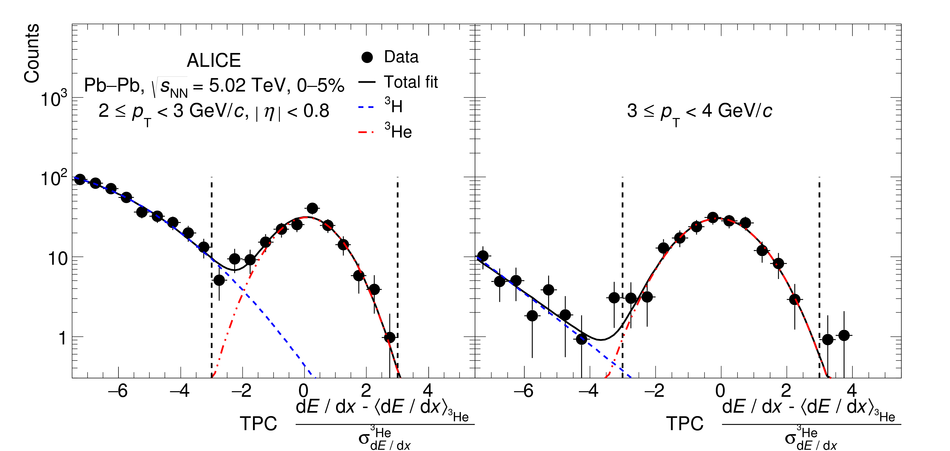## Figure 2

 c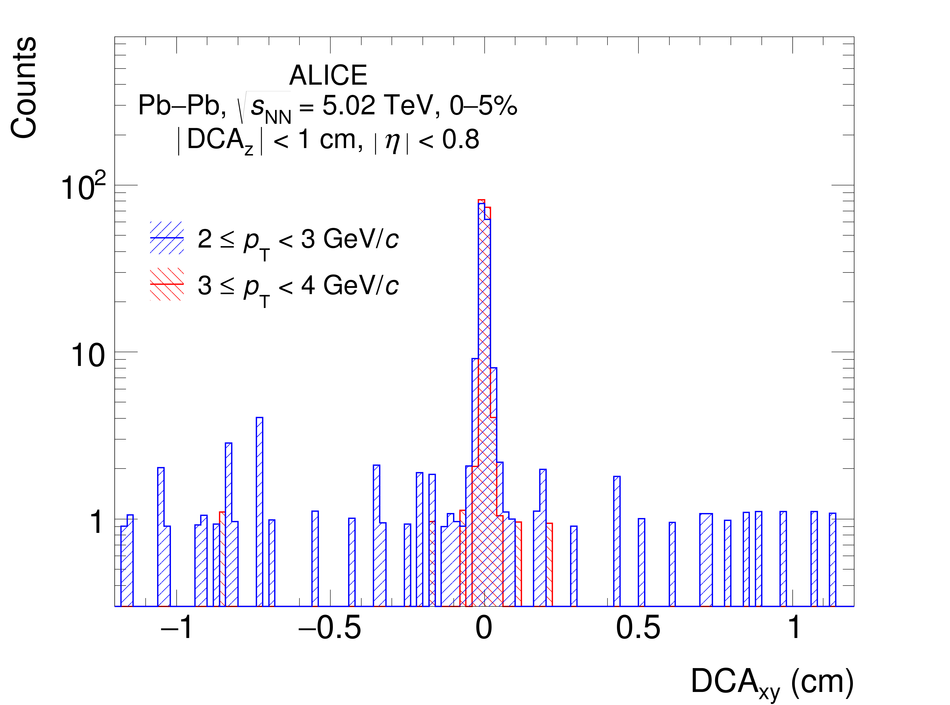## Figure 3

 Event-plane resolution $R_{\Psi_{2}}$ of the second harmonic as a function of the collision centrality.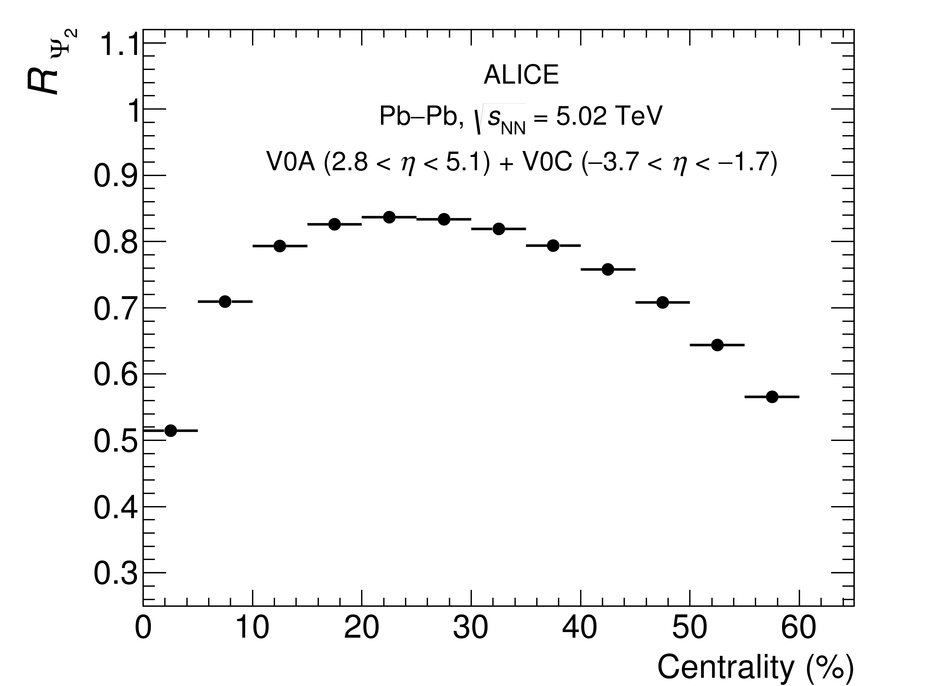## Figure 4

 Elliptic flow ($v_{2}$) of (anti-)$^{3}$He measured in \PbPb collisions at \snn = 5.02 TeV for the centrality classes 0--20$\%$, 20--40$\%$, and 40--60$\%$. The statistical uncertainties are shown as vertical bars, systematic uncertainties as boxes.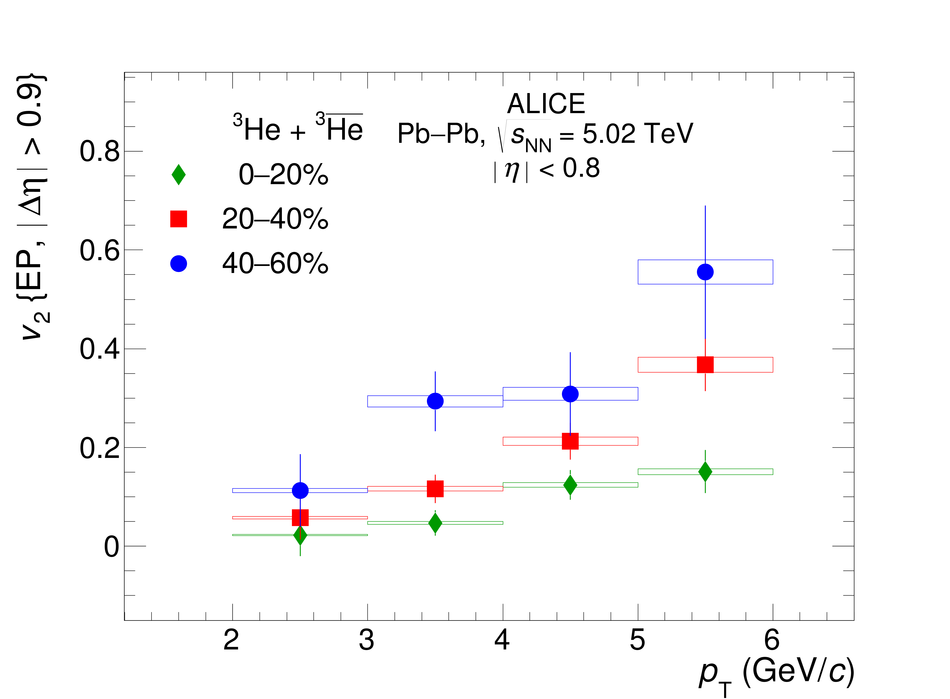## Figure 5

 Comparison between the elliptic flow of (anti-)$^{3}$He measured using the event-plane method and that of pions, kaons, and protons measured using the scalar-product method in \PbPb collisions at \snn = 5.02 TeV for the centrality classes 0--20$\%$ (left), 20--40$\%$ (middle) and 40--60$\%$ (right). See text for details. Vertical bars and boxes represent the statistical and systematic uncertainties, respectively.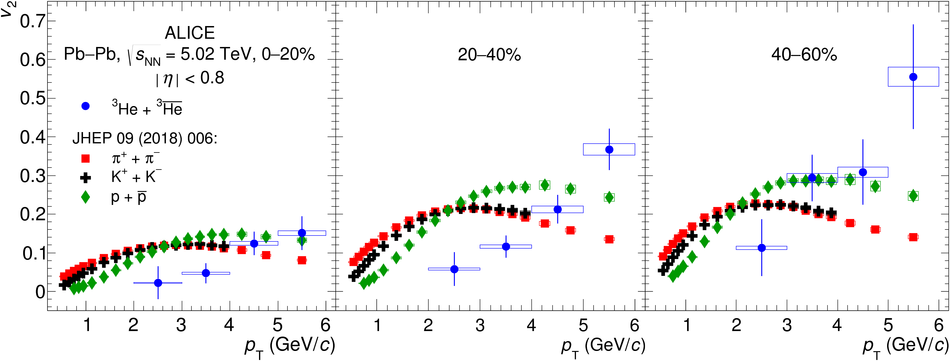## Figure 6

 Comparison between the elliptic flow of pions, kaons, protons, and (anti-)$^{3}$He divided by the number of constituent quarks ($n_{\mathrm{q}}$) as a function of $p_{\mathrm{T}}/n_{\mathrm{q}}$ (upper panels) and transverse kinetic energy per constituent quark $E^{\mathrm{kin}}_{\mathrm{T}}/n_{\mathrm{q}}$ (lower panels) for the centrality classes 0--20$\%$ (left), 20--40$\%$ (middle) and 40--60$\%$ (right). See text for details. Vertical bars and boxes represent the statistical and systematic uncertainties, respectively.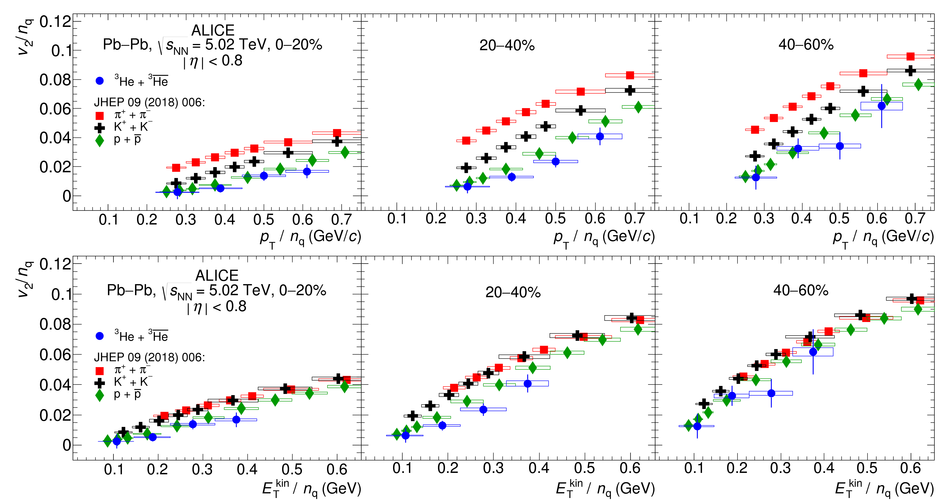## Figure 7

 Elliptic flow of (anti-)$^{3}$He in comparison with the predictions from the Blast-Wave model and a simple coalescence approach for the centrality classes 0--20$\%$ (left), 20--40$\%$ (middle), and 40--60$\%$ (right). The lower panels show the differences between data and models for each centrality range. The statistical uncertainties of the data and the model are added in quadrature. Vertical bars and boxes represent the statistical and systematic uncertainties, respectively.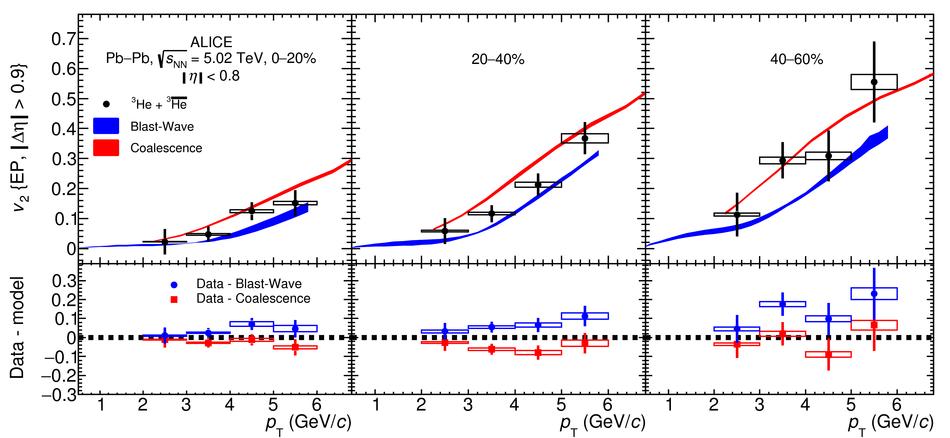## Figure 8

 Elliptic flow of (anti-)$^{3}$He measured in the centrality classes 0--20$\%$ and 20--40$\%$ in comparison with the predictions from a coalescence model based on phase-space distributions of protons and neutrons generated from the iEBE-VISHNU hybrid model with AMPT initial conditions . The model predictions are shown as lines and the bands represent their statistical uncertainties. The differences between data and model are shown in the lower panel for both centrality classes. The statistical uncertainties of the data and the model are added in quadrature. Vertical bars and boxes represent the statistical and systematic uncertainties, respectively.